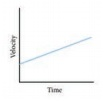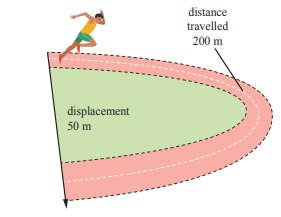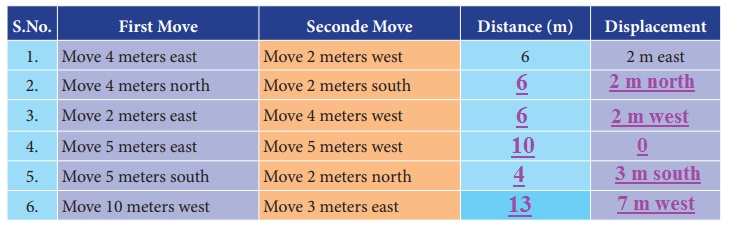Home | | Science 7th Std | Questions Answers

## Chapter: 7th Science : Term 1 Unit 2 : Force and Motion

7th Science : Term 1 Unit 2 : Force and Motion : Book Back Questions Answers, Solution

Evaluation

1. A particle is moving in a circular path of radius r. The displacement after half a circle would be

a. Zerob.

b. R

c. 2 r.

d. r / 2

2. Which of the following figures represent uniform motion of a moving object correctly?3. Suppose a boy is enjoying a ride on a marry go round which is moving with a constant speed of 10 m/s. It implies that the boy is

a. at rest

b. moving with no acceleration

c. in accelerated motion

d. moving with uniform velocity

Answer : c. in an accelerated motion

4. From the given v-t graph it can be inferred that the object isa. in uniform motion

b. at rest

c. in non uniform motion

d. moving with uniform acceleration

Answer : d. moving with uniform acceleration

5. How can we increase the stability of an object?

a. lowering the centre of gravity

b. raise the centre of gravity

c. increasing the height of the object

d. shortening the base of the object

Answer : a. lowering the centre of gravity

II. Fill in the blanks.

1. The shortest distance between the two places is displacement.

2. The rate of change of velocity is acceleration.

3. If the velocity of an object increases withrespect to time, then the object is said to be in positive acceleration.

4. The slope of the speedŌĆōtime graph gives acceleration of a body.

5. In neutral equilibrium its centre of gravity remains at the same height when it is displaced.

III. Match the following:

Displacement                                            -                Knot

Light travels through vacuum                   -                Geometric centre

Speed of ship                                             -                Metre

Centre of gravity of the geometrical shaped object  -   Larger base area

Stability                                                    -                  Uniform velocity

1. Displacement                            -    Metre

2. Light travels through vacuum   -    Uniform velocity

3. Speed of ship                               -   Knot

4. Centre of gravity of the geometrical shaped object- Geometric centre

5. Stability                                        -  Larger base area

IV. Analogy

1. velocity : metre/ second : : acceleration : meter / second2.

2. length of scale : metre : : speed of aeroplane: knot.

3. displacement / time : velocity : : speed / time: acceleration.

1. All objects having uniform speed need not have uniform velocity. Describe with the help of examples.If an athlete in the diagram takes 25 s to complete a 200 m sprint event. Then

Speed = distance / time

=  200 / 25

= 8 m / s

He travels with an uniform speed since he covers equal distances in equal intervals of time.

velocity = displacement / time

= 50 / 25

= 2 m/s

Ōł┤ Velocity is not uniform because there is change in direction.

2. ŌĆ£She moves at a constant speed in a constant directionŌĆØ. Rephrase the same sentence in fewer words using concepts related to motion.

Saphira moves with a uniform velocity.

3. Correct your friend who says ŌĆ£The acceleration gives the idea of how fast the position changesŌĆØ.

The acceleration gives the idea of change of velocity with time.

1. Show the shape of the distance ŌĆō time graph for the motion in the following cases.

a. A bus moving with a constant speed.b. A car parked on a road side.2. Distinguish between speed and velocity.Speed (m/s)

1. It is the distance travelled per unit time.

2. It is a scalar quantity

3. It is the change in distance divided by change in time.

4. It has only magnitude.

5. Speed always positive whether it is moving to right or left.

6. Megnitude of speed in no way equal to Zero.

Velocity (m/s)

1. It is the distance travelled in a particular direction per unit time.

2. It is a vector quantity

3. It is the change in displacement divided by change in time.

4. It has both magnitude and direction.

5. Velocity is positive if it moves to right and it is negative it is moves to left.

6. Magnitude of velocity may be zero.

3. What do you mean by constant acceleration?

An object undergoes uniform (constant) acceleration when the change (increase or decrease) in its velocity for every unit of time is the same. Table shows a moving bus with uniform acceleration.When the velocity of the object is increasing by 20 m/s the acceleration is 20 m/s2. When the velocity of the object is decreasing by 20 m/s the deceleration is 20 m/s2.

When the velocity of the object is decreasing by 20m/s the deceleration is 20 m/s2.

Deceleration = Decrease in velocity / Deceleration = t = 20/20 = 1s

The velocity of the object is decreasing by 20m/s in one second.

4. What is centre of gravity ?

The centre of gravity of an object is the point through which the entire weight of the object appears to act.

1. Explain the types of stability with suitable examples.

Stability is a measure of the body's ability to maintain its original position.

(a) Stable equilibrium

(b) Unstable equilibrium

(c) Neutral equlibrium

Stable Equlibrium :

The frustum can be tilted through quite a big angle without toppling. Its centre of gravity is raised when it is displaced.

The vertical line thorugh its centre of gravity still falls within its base. So it can return to its original position.Unstable Equilibrium :

The frustum will topple with the slightest tilting. Its centre of gravity is lowered when it is displaced.

The vertical line through its centre of gravity falls outside its base.ŌĆāNeutral Equilibrium :

(d) It does not cause to topple.

(e) The frustum will rolls about but does not topple.

(f) Its centre of gravity ramains at the same height when it it displaced.

(g) The body will stay in any position to which it has been displaced.2. Write about the experiment to find the centre of gravity of the irregularly shaped plate.

Determination of centre of gravity of irregular shaped objects.

Apparatus : Irregularly shaped plate string, pendulum bob, stand.1. Make three holes in the lamina.

2. Suspend the lamina from the optical pin through one of the holes as shown.

3. Suspend the plumbline from the pin and mark and position of the plumbline on the lamina.

4. Draw lines on the lamina representing the positions of the plumbline.

5. Repeat the above steps for the holes.

6. Label the intersection of the three lines as X, the position of the centre of gravity of the lamina.

VIII. Numerical problems.

1. Geetha takes 15 minutes from her house to reach her school on a bicycle. If the bicycle has a speed of 2 m/s, calculate the distance between her house and the school.

Time taken by Geetha = 15 minutes

= 15 ├Ś 60 seconds

= 900 s

Her speed                     = 2 m/s

Distanced moved by her in 1 s = 2 m

Ōł┤  in 900 s                      = (900 s / 1 s) ├Ś 2 m

= 1800 m = 1.8 km

2. A car started from rest and travelling with velocity of 20 m /s in 10 s. What is its acceleration?

Initial velocity (U) = 0 m /s

Final Velocity (V)  = 20 m /s

Change in Velocity = (V - U) = (20 ŌĆō 0 ) = 20 m/s

Time taken for this change in velocity = 10 s

Acceleration = change in velocity / time

a = 20 m/s / 10 s = 2 m/s2

3.A bus can accelerate with an acceleration 1 m / s2. Find the minimum time for the bus to reach the speed of 100 km / s from 50 km / s.

Acceleration of the bus a   = 1 m/s2

Change in speed = (V - U) = (100 - 50) km / hr

= 50 ├Ś 1000 m/hr

= (50000 / 60) m/ minute

= (50,000 / 60├Ś60 ) m/second

Time taken for this change of speedŌĆā= change in speed / acceleration

= [ 50,000 m/s ] / [ 60 ├Ś 60 ├Ś 1 ms2] = 125 /9 s

= 13.89 seconds

IX. Fill in the boxes.Tags : Force and Motion | Term 1 Unit 2 | 7th Science , 7th Science : Term 1 Unit 2 : Force and Motion
Study Material, Lecturing Notes, Assignment, Reference, Wiki description explanation, brief detail
7th Science : Term 1 Unit 2 : Force and Motion : Questions Answers | Force and Motion | Term 1 Unit 2 | 7th Science